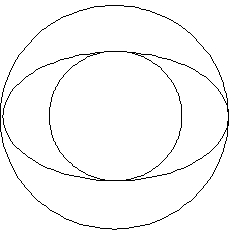#### You may also like### Gold Again

Without using a calculator, computer or tables find the exact values of cos36cos72 and also cos36 - cos72.### Pythagorean Golden Means

Show that the arithmetic mean, geometric mean and harmonic mean of a and b can be the lengths of the sides of a right-angles triangle if and only if a = bx^3, where x is the Golden Ratio.### Golden Triangle

Three triangles ABC, CBD and ABD (where D is a point on AC) are all isosceles. Find all the angles. Prove that the ratio of AB to BC is equal to the golden ratio.

# Golden Eggs

##### Age 16 to 18 Challenge Level:1) An ellipse with semi axes $a$ and $b$ fits between two circles of radii $a$ and $b$ (where $b> a$) as shown in the diagram. If the area of the ellipse is equal to the area of the annulus what is the ratio $b:a$?

(2) Find the value of $R$ if this sequence of 'nested square roots' continues indefinitely: $$R=\sqrt{1 + \sqrt{1 + \sqrt {1 + \sqrt {1 + ...}}}}.$$